## Example Questions

### Example Question #601 : Arithmetic

Find the common difference of the following sequence: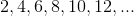Explanation:

The common difference is equal to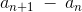.

Plugging in the values from this problem we get,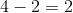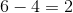Therefore, in this case the common difference is.

### Example Question #1671 : Act Math

The following is an arithmetic sequence. Find an explicit equation for it in terms of the common difference.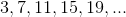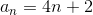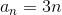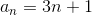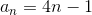Explanation:

Finding the common difference is fairly simple. We simply subtract the first term from the second. 7-3 = 4, so 4 is our common difference. So each term is going to be 4n plus something: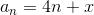We know the first term is 3, so we can plug in that to our equation.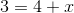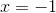So the explicit form of our arithmetic sequence is.

### Example Question #1 : Arithmetic Sequences

Which of the following completes the number sequence 4, 7, 11, 16, __________ ?Explanation:

Sequencing problems require us to look at the numbers given to us ad decipher a pattern.

7  4 = 3 so 3 was added to the first number (4)

11 – 7 = 4 so 4 was added to the second number (7)

16  11 = 5 so 5 was added to the third number (11)

If it is to continue in this pattern, then 6 should be added to 16, yielding 22 as the correct answer

### Example Question #1 : Arithmetic Sequences

Find the 50th term in the following sequence.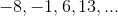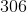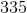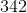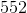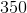Explanation:

A sequence is simply a list of numbers that follow some sort of consistent rule in getting from one number in the list to the next one.  Sequences generally fall into three categories:  arithmetic, geometric, or neither.

In arithmetic sequences, I add the same number each time to get from one number to the next.  In other words, the difference between any two consecutive numbers in my list is the same.

In geometric sequences, I multiply by the same number each time to get from one number to the next.  In other words, the ratio between any two consecutive numbers in my list is the same.

Finally, sequences that are neither, still follow some rule, but it just happens not to be one of these two.

Looking at our sequence, we might quickly notice that each number is simply 7 more than the number before.  In other words, I can find the next number by adding 7 each time.  Hence, our sequence is arithmetic.

Unfortunately, we need to find the 50th term in this sequence, and the problem only got us through the first four.  A simple (yet way too time-consuming approach) would be to keep adding 7 until we get to term number 50.  Not only is that the long way, we also risk losing count and ending up on the wrong term.  So what's the easier way?

The easier way hinges on the fact that I am simply adding 7 over and over again.  If I want to find the 2nd term, I start with the 1st term and add 7 once.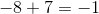To find the 3rd term, I add 7 twice.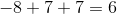You might already see the pattern.  For the 4th term I would add 7 three times, for the 5th four times, 6th five times, etc.

Notice that to find any term, I simply add 7 one less time than the number of the term.  Therefore, to find the 50th term, I would add 7 forty-nine times.

But adding 7 forty-nine times is the same as adding forty-nine 7s.  But forty-nine 7s are the same as 49 times 7.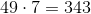Therefore, to find the 50th term, I simply need to add 343 to our starting value.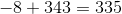### Example Question #3 : Arithmetic Sequences

What is the next term in the sequence: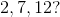Explanation:

Because it is an arithmetic sequence, the difference between each term is the same. Therefore find out the difference between any two consecutive terms.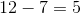and so the sequence increases by 5 each term. Thus the answer is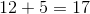.

### Example Question #4 : Arithmetic Sequences

The sum of four consecutive integers is 42. What is the value of the greatest number?

12

15

13

14

11

12

Explanation:

We can represent four consecutive integers using the following expressions:Create an equation using the information in the problem.Subtract 6 from both sides of the equation.Divide both sides of the equation by 4.The highest number in the series is the x-variable plus 3. We can write the following: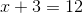.

### Example Question #1 : Arithmetic Sequences

Which of the following numbers completes the sequence 40, 33, 27, 22, 18, 15, ...?

13

7.5

14

16

12

13

Explanation:

To get the next term in the sequence you subtract a decreasing amount from the preceding term. You subtract 7 from 40 to get 33, then 6 from 33 to get 27, and so on until you subtract 2 from 15 to get 13.

### Example Question #1 : Arithmetic Sequences

If the first day of the year is a Monday, what is the 295th day?

Saturday

Monday

Tuesday

Wednesday

Monday

Explanation:

The 295th day would be the day after the 42nd week has completed. 294 days/7 days a week = 42 weeks. The next day would therefore be a monday.

### Example Question #1 : How To Find The Nth Term Of An Arithmetic Sequence

If the first two terms of a sequence are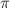and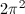, what is the 38th term?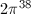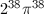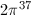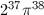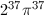Explanation:

The sequence is multiplied by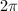each time.

### Example Question #1 : Arithmetic Sequences

Find the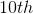term of the following sequence: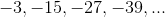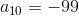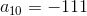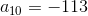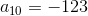Explanation:

The formula for finding the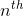term of an arithmetic sequence is as follows: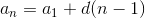where= the difference between consecutive terms= the number of terms

Therefore, to find theterm: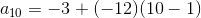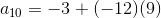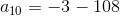### All ACT Math Resources